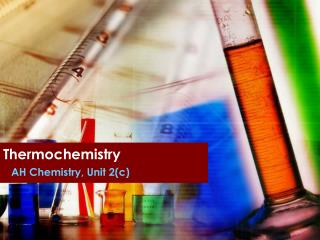DownloadDownload PresentationThermochemistry

# Thermochemistry

Download Presentation## Thermochemistry

- - - - - - - - - - - - - - - - - - - - - - - - - - - E N D - - - - - - - - - - - - - - - - - - - - - - - - - - -
##### Presentation Transcript

1. Thermochemistry AH Chemistry, Unit 2(c)

2. Introduction • Thermochemistry concerns the study of energy changes in chemical reactions. • First Law of Thermodynamics: “Energy is conserved”. • Hess’s law states that “the overall reaction enthalpy is the sum of the reaction enthalpies of each of step of a reaction”.

3. Calorimetry • The term used to describe the quantitative determination of the change in heat energy which occurs during a chemical reaction. • A calorimeter is used to measure this energy change.

4. “Standard” enthalpy changes • Both reactants and products are considered in their most stable state at 1 atmosphere pressure and a specified temperature (usually 298K).

5. Standard Enthaply of Formation • Refers to the enthalpy change which occurs when one mole of a substance is prepared from its elements in their standard state.

6. Examples C(s) + O2(g) ———> CO2(g) H2(g)+½O2(g) ———>H2O(l) 2C(s) + ½O2(g) + 3H2(g) ———>C2H5OH(l) Notes Only ONE MOLE of product on the RHS of the equation. Elements In their standard states have zero enthalpy of formation. Carbon is usually taken as the graphite allotrope.

7. Using enthalpies of formation Example Calculate the standard enthalpy of reaction at 298K for the complete combustion of methane. Hº =  Hf (products) – Hf (reactants) -891.1 kJ mol l-1

8. Bond Enthalpies

9. Born-Haber cycles

10. What are they? • A thermochemical cycle applied to the formation of an ionic crystal. • Used to calculate the enthalpy of lattice formation, which cannot be determined directly by experiment. • Standard Enthalpy of Lattice Formation:the enthalpy change which occurs when one mole of an ionic crystal is formed from the ions of their gaseous states, under standard conditions.

11. Na+ (g) + e- + Cl (g) H first ionisation energy H first electron affinity Na (g) + Cl (g) Na+ (g) + Cl-(g) H bond enthalpy Na (g) + ½Cl2 (g) H lattice H atomisation Na (s) + ½Cl2 (g) H formation NaCl (s) Born-Haber cycle

12. Na+ (g) + e- + Cl (g) enthalpy H H first ionisation energy H first electron affinity Na (g) + Cl (g) Na+ (g) + Cl-(g) H atomisation Na (g) + ½Cl2 (g) H lattice H atomisation Na (s) + ½Cl2 (g) H formation NaCl (s) Apply Hess’s Law: Hformation HatmNa + HatmCl + H1st IE + H1st EA + Hlattice =

13. Born-Haber Cycles: applying Hess’s Law Hformation HatmNa + HatmCl + H1st IE + H1st EA + Hlattice = Rearrange to find the lattice energy: Hlattice = Hformation - (HatmNa + HatmCl + H1st IE + H1st EA) So Born-Haber cycles can be used to calculate a measure of ionic bond strength based on experimental data.

14. Definitions Standard enthalpy of atomisation of an element: The energy required to produce one mole of isolated gaseous atoms from the element in its standard state. Electron affinity: The enthalpy change for the process of adding one mole of electrons to one mole of isolated atoms in the gaseous state.

15. Enthalpy of solution • Energy must be supplied to break up the ionic lattice: ∆Hlatt • Energy is released when the free ions form bonds with water molecules: ∆Hhyd Hydration enthalpy: The energy released when one mole of individual ions become hydrated.

16. Illustrate the hydration enthalpy for: • A sodium ion • An sulphate ion